# Unions of graphs

Relevant articles: , , , , , , , , , , , , , , , , , , .

[hide]

# Unions of graphs

In this section we consider the existence of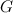$G$-designs for cases where$G$ is the union of pairwise vertex-disjoint graphs, or graphs intersecting in only one vertex. Many such results can be obtained indirectly via solutions to other related problems, and yield only a few scattered existence results on the spectrum problem. A description and summary of all such results would be very lengthy, full of partial results, and not really in line with the focus of this website.

The survey article 'A Survey on the Existence of$G$-Designs' by Adams, Bryant and Buchanan  briefly discuss a few examples to indicate the types of results that can be obtained in this manner.

## Spectrum Results

An explanation of the sources of most of the follwoing results is given in , and the remainder is a correction to an error in  as discussed below.

There is an error in the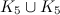$K_5\cup K_5$-design of order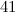$41$ given in Example A.28 in the Appendix of . Instead, let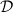$\cal D$ consist of the orbit of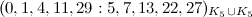$(0,1,4,11,29 : 5,7,13,22,27)_{K_5\cup K_5}$ under the permutation$\rho_{41}$. Then$\cal D$ is a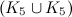$(K_5\cup K_5)$-design of order$41$.

Theorem 1

Denote by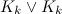$K_k\vee K_k$ the graph consisting of two copies of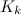$K_k$ sharing a vertex. There exists a$K_k\vee K_k$-design of order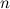$n$ if and only if there exists a$K_k$-design of order$n$ and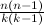${n(n-1) \over k(k-1)}$ is even.

Combining Theorem 1 with the results given in Table 1 in the section on Complete Graphs we have a complete solution to the spectrum problem for$K_k\vee K_k$ when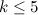$k\leq 5$, see Table 1 below.

Table 1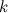$k$ Spectrum for$K_k\vee K_k$ Possible exceptions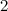$2$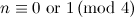$n \equiv 0 {\rm \ or \ } 1 \,({\rm mod \ }4)$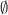$\emptyset$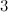$3$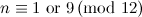$n \equiv 1 {\rm \ or \ } 9 \,({\rm mod \ }12)$$\emptyset$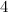$4$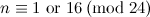$n \equiv 1 {\rm \ or \ } 16 \,({\rm mod \ }24)$$\emptyset$$5$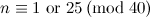$n \equiv 1 {\rm \ or \ } 25 \,({\rm mod \ }40)$$\emptyset$

Theorem 2

Denote by$\cup^mK_k$ the graph consisting of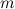$m$ vertex disjoint copies of$K_k$. If there exists a$K_k$-design of order$n$ and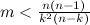$m<{n(n-1) \over k^2(n-k)}$ then there exists a$\cup^mK_k$ design of order$n$.

We can use Theorem 2 and some small designs given in  to settle the spectrum problem for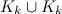$K_k\cup K_k$ when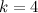$k=4$ and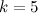$k=5$. The cases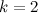$k=2$ and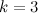$k=3$ are also settled, see the section on matchings or the section on small graphs for$k=2$ and the section on even graphs for$k=3$. We summarise these results in Table 2 below.

Table 2$k$ Spectrum for$K_k\cup K_k$ Exceptions$2$$n \equiv 0 {\rm \ or \ } 1 \,({\rm mod \ }4)$$\emptyset$$3$$n \equiv 1 {\rm \ or \ } 9 \,({\rm mod \ }12)$${\rm There \ is \ no \ }K_3 \cup K_3{\rm -design \ of \ order \ } 9.$$4$$n \equiv 1 {\rm \ or \ } 16 \,({\rm mod \ }24)$$\emptyset$$5$$n \equiv 1 {\rm \ or \ } 25 \,({\rm mod \ }40)$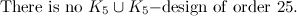${\rm There \ is \ no \ }K_5 \cup K_5{\rm -design \ of \ order \ } 25.$

## Notes

• Results on the Oberwolfach problem yield$G$-designs of$K_n$ in the case$G$ is a$2$-regular graph on$n$ vertices. A table of known results on the Oberwolfach problem is given in .
• Various small disconnected graphs and small graphs with cut-vertices which could be mentioned in this section are covered in the section on small graphs.

## References

1. Abel, R. J. R., Ge, G., and Yin, J. Resolvable and Near-Resolvable Designs, CRC Press Series on Discrete Mathematics and its Applications, The CRC handbook of combinatorial designs, ed. 2, CRC Press, 124–132 (2007).
2. Adams, P., Ardal, H., Maňuch, Ján., Hòa, V. D., Rosenfeld, M., and Stacho, L. Spanning cubic graph designs, Discrete Math. 309, 5781–5788 (2009).
3. Adams, P. and Bryant, D. Two-factorisations of complete graphs of orders fifteen and seventeen, Australas. J. Combin. 35, 113–118 (2006).
4. Adams, P., Bryant, D. E., and Khodkar, A. Uniform 3-factorisations of K_10, Proceedings of the Twenty-eighth Southeastern International Conference on Combinatorics, Graph Theory and Computing (Boca Raton, FL, 1997), 23–32 (1997).
5. Adams, P., Bryant, D., and Maenhaut, B. Cube factorizations of complete graphs, J. Combin. Des. 12, 381–388 (2004).
6. 6.0 6.1 6.2 6.3 6.4 6.5 Adams, P., Bryant, D., and Buchanan, M. A survey on the existence of G-designs, J. Combin. Des. 16, 373–410 (2008).
7. Alspach, B., Schellenberg, P. J., Stinson, D. R., and Wagner, D. The Oberwolfach problem and factors of uniform odd length cycles, J. Combin. Theory Ser. A, 52, 20–43 (1989).
8. Bermond, J. -C., Heinrich, K., and Yu, M. Existence of resolvable path designs, European J. Combin. 11, 205–211 (1990).
9. Bryant, D. and El-Zanati, S. Graph decompositions, CRC Press Series on Discrete Mathematics and its Applications, The CRC handbook of combinatorial designs, ed. 2, CRC Press, 477–486 (2007).
10. 10.0 10.1 Bryant, D. and Rodger, C. A. Cycle decompositions, CRC Press Series on Discrete Mathematics and its Applications, The CRC handbook of combinatorial designs, ed. 2, CRC Press, 373–382 (2007).
11. Colbourn, C. J., Stinson, D. R., and Zhu, L. More frames with block size four, J. Combin. Math. Combin. Comput. 23, 3–19 (1997).
12. Hajnal, A. and Szemerédi, E. Proof of a conjecture of P. Erdös, Combinatorial theory and its applications, II (Proc. Colloq., Balatonfüred, 1969), North-Holland, Amsterdam, 601–623 (1970).
13. Hanani, H., Ray-Chaudhuri, D. K., and Wilson, R. M. On resolvable designs, Discrete Math. 3, 343–357 (1972).
14. Horák, P. and Rosa, A. Decomposing Steiner triple systems into small configurations, Ars Combin. 26, 91–105 (1988).
15. Huang, C. Resolvable balanced bipartite designs, Discrete Math. 14, 319–335 (1976).
16. Lonc, Z. On resolvable tree-decompositions of complete graphs, J. Graph Theory, 12, 295–303 (1988).
17. Ray-Chaudhuri, D. K. and Wilson, R. M. Solution of Kirkman's schoolgirl problem, Combinatorics (Proc. Sympos. Pure Math., Vol. XIX, Univ. California, Los Angeles, Calif., 1968), Amer. Math. Soc. Providence, R.I. 187–203 (1971).
18. Yu, M. On tree factorizations of K_n, J. Graph Theory, 17, 713–725 (1993).
19. Yu, M. Almost resolvable P_k-decompositions of complete graphs, J. Graph Theory, 15, 523–541 (1991).